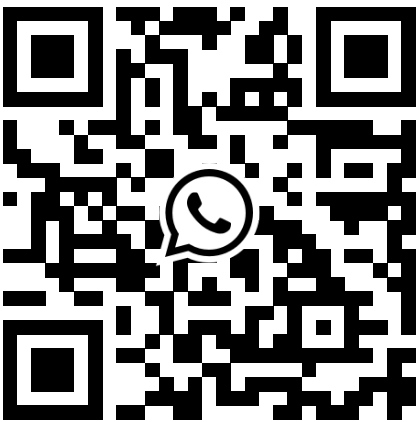﻿ What Do These Parameters Represent in the Din Energy Meter? - Acrel Co., Ltd.
English

# What Do These Parameters Represent in the Din Energy Meter?

Electric meter is short for din energy meter, which is an instrument used to measure electric energy. It is also called an electricity meter, fire meter, electricity meter, kilowatt hour meter, which measures various electrical quantities.

## Identification of din energy meter

The identification and differentiation of electric meters are based on national standard GB/T15283-94 and international standard IEC521-1988. The din energy meter has two current values marked, such as 10 (20) A. The marked 10A is the basic current, which is the current value that determines the characteristics of the meter and is also called the calibration current. The value in parentheses, 20A, is the rated maximum current, which is the maximum current value that the meter can meet the standard accuracy requirements.

## Basic understanding of the accuracy of the din energy meter

The current through the din energy meter can reach 2-8 times the basic current value, and if it does not reach 2 times, only the basic current value is marked on the meter. That is to say, if a din energy meter installed by a user only has one current value marked, such as 5A, this is only the basic current value and not the maximum allowable current. For this kind of din energy meter, it can generally be overloaded up to 120% without any problems, and can meet accurate measurement of din energy meter.

On the other hand, due to the large resistance of the induction system of the din energy meter, the starting current must not be lower than 0.5% of the basic current (for level accuracy din energy meters) according to the standard requirements. Therefore, the din energy meter may not start when the light load drops below 0.5% of the basic current value.

In Chinese residential power supply lines, the voltage is 220V and the frequency is 50Hz. The rated voltage and applicable frequency of the selected din energy meter should be consistent with this line voltage and frequency, which should also be 220V, 50Hz.

## Selection of the din energy meter

When selecting din energy meters, the current value selection is the most important and most complex.

One is the starting current, which is the minimum current that can make the disc rotate continuously; the other is the multiple of the maximum rated current relative to the basic current. In addition, there are differences in performance between old and new models.

The old-style din energy meter still used in old houses has a relatively large starting current, generally around 5%-10%; the maximum rated current is small, generally ≤2, and only one current value is marked on the dial surface, which is roughly referred to as the rated current. Therefore, the old electrician's manual indicates that the current of the load circuit should be greater than 10% of the rated current and less than 120% or less than 125% when used.

The din energy meter manufactured according to the national standard GB/T15283-94 and international standard IEC521-1988 used in newly-built houses have a smaller starting current, which is 0.5% for graded meters; the maximum rated current is large, generally between (2-4), and some can reach (6-8).•